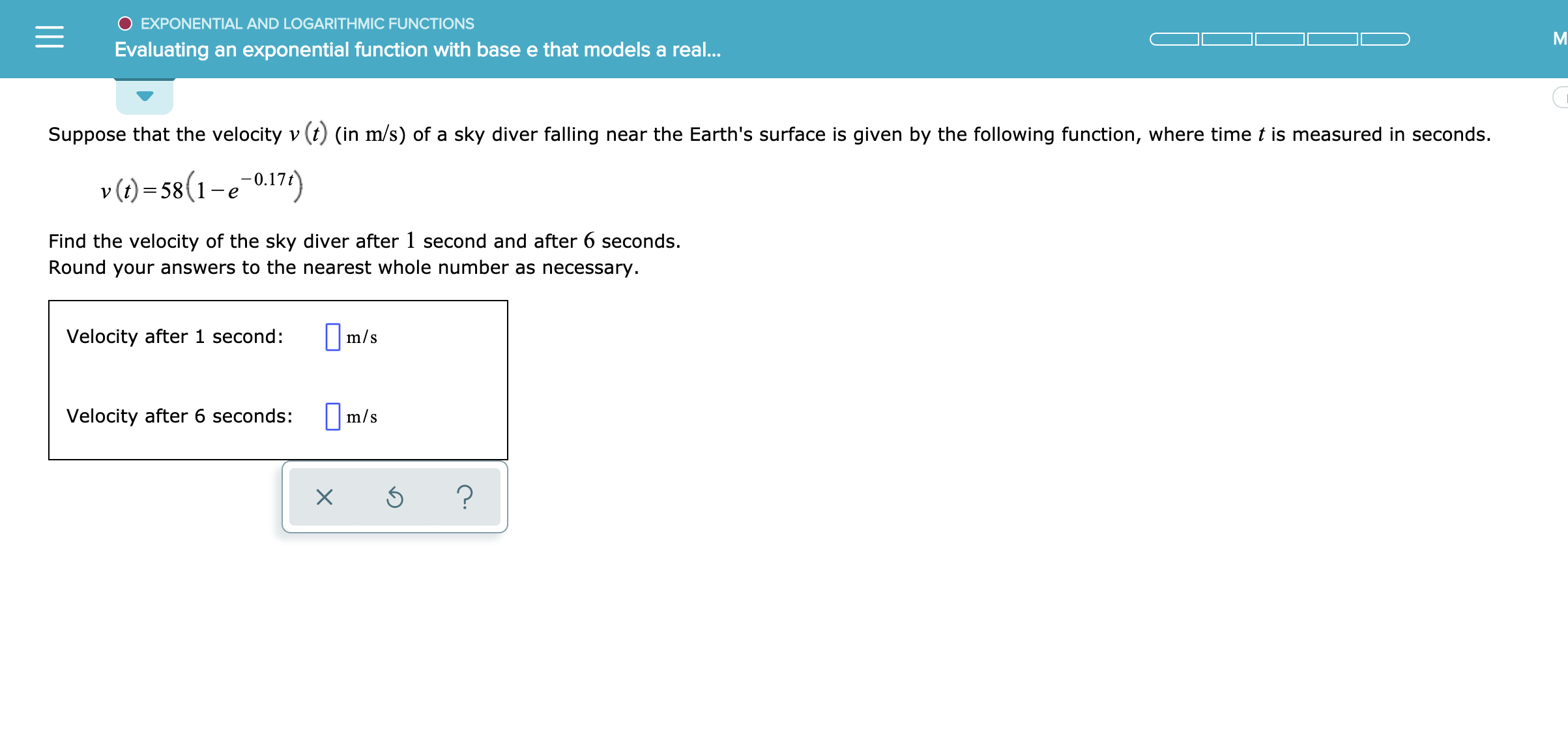# O EXPONENTIAL AND LOGARITHMIC FUNCTIONS Evaluating an exponential function with base e that models a real... Suppose that the velocity v (t) (in m/s) of a sky diver falling near the Earth's surface is given by the following function, where time t is measured in seconds. )58(1-e0.17) _ Find the velocity of the sky diver after 1 second and after 6 seconds. Round your answers to the nearest whole number as necessary |m/s Velocity after 1 second: m/s Velocity after 6 seconds: ?

Question

see attachedhelp_outlineImage TranscriptioncloseO EXPONENTIAL AND LOGARITHMIC FUNCTIONS Evaluating an exponential function with base e that models a real... Suppose that the velocity v (t) (in m/s) of a sky diver falling near the Earth's surface is given by the following function, where time t is measured in seconds. )58(1-e0.17) _ Find the velocity of the sky diver after 1 second and after 6 seconds. Round your answers to the nearest whole number as necessary |m/s Velocity after 1 second: m/s Velocity after 6 seconds: ? fullscreen

## Expert Answer

### Want to see this answer and more?

Experts are waiting 24/7 to provide step-by-step solutions in as fast as 30 minutes!*

*Response times may vary by subject and question complexity. Median response time is 34 minutes for paid subscribers and may be longer for promotional offers.
Tagged in
Math
Calculus

### Functions© 2021 bartleby. All Rights Reserved.